# Mathematical problems for self assessment

###### LAPLACE TRANSFORMS

1. Show from first principles (by using integration by parts) that the Laplace transform ofis given by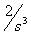.

2. Find (from tables) the Laplace transforms of the following functions: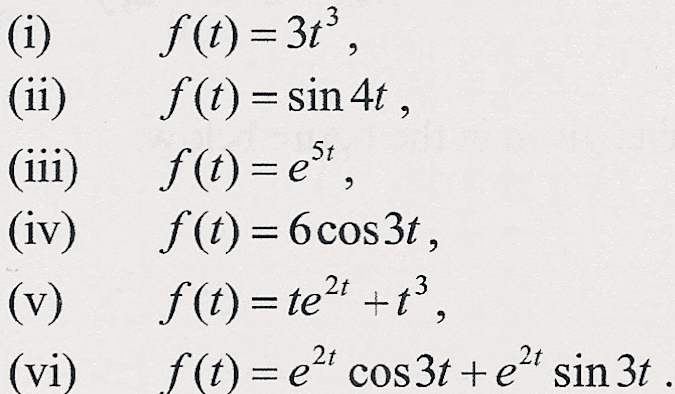[Ans.].

3. Find (from tables) the inverse Laplace transforms of the following functions:

(i)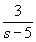,

(ii),

(iii)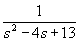,

(iv),

(v),

(vi)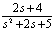.

[Ans. (i)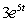, (ii), (iii)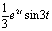, (iv)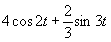, (v)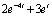, (vi)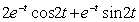].

4. Find the Laplace transforms of the following expressions:

(i)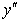;,

(ii)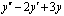;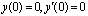,

(iii)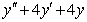;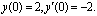[Ans. (i), (ii)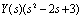, (iii)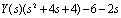, where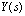is the Laplace transform of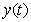].

5. Solve the following differential equation using Laplace transforms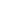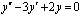,.

[Ans.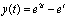].

6. Solve the following differential equation using Laplace transforms,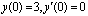.

[Ans.].

7. Solve the following differential equation using Laplace transforms,.

[Ans.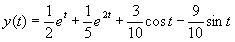].

8. Solve the following differential equation using Laplace transforms,.

[Ans.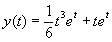].

9. Solve the following differential equation using Laplace transforms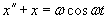,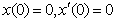,

for some constant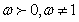.

[Ans.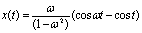].